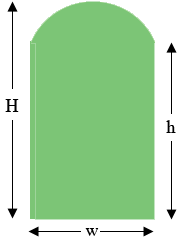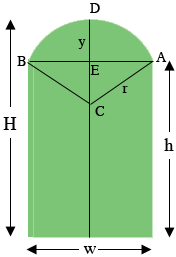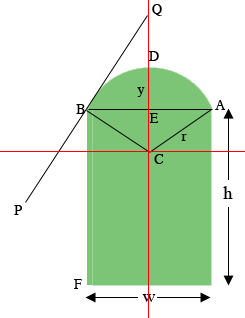SEARCH HOMEMath Central Quandaries & QueriesQuestion from Richard: Hello, I was hoping your math specialists could help me with some formulas. I have shapes with specific known variables need to calculate others.   Example: We make a straight legged arch, This shape has a width a overall height and a leg height, The leg height is always less then the overall, And the top is arched. We have the width, Height, and leg size.   Need to calculate the length of the curve and sq ft of the shape.   If you guys can help let me know, I can send drawings with given information.Hi Richard,Does the diagram above depict the situation you described, where the width $w,$ the overall height $H,$ and the leg length $h,$ are known? I am assuming the arch is a section of a circle. If so can you send me $w, H$ and $h$ for a particular example and I will generate general expressions for you and use the dimensions you send for an example calculation.

Harley

Richard replied

Yes your diagram is perfect.
H = 71.5
h = 62
w = 35.5

I redid the diagram and added to it. $C$ is the center of the circle that forms the arch, $r$ is the radius of the circle, $E$ is the midpoint of the line segment $AB,$ and $y = H - h.$I assume your measurements are in inches.

The first task is to find the value of $r.$ Triangle $AEC$ is a right triangle so Pythagoras Theorem gives

$|CA|^2 = |AE|^2 + |EC|^2 \mbox{ or } r^2 = \left( \frac{w}{2} \right)^2 + (r-y)^2$

solving this expression for $r$ and simplifying gives

$r = \frac{4 y^2 + w^2}{8 y} \mbox{ units.}$

For the example you sent this gives

$r = \frac{4 \times (71.5 - 62)^2 + 35.5^2}{8 \times (71.5 - 62} = 21.33 \mbox{ inches.}$

I next need to know the measure of the angle $ACB.$ Again from triangle $AEC,$

$\sin \left( ACE \right) = \frac{|AE|}{|CA|} = \frac{w/2}{r}$

and hence the measure of angle $ACE$ is

$\sin^{-1}\left( \frac{w/2}{r} \right).$

$sin^{-1}$ is sometimes written $\arcsin$ or ASIN.

Thus the measure of the angle $ACB,$ I'm going to call it $\theta,$ is

$\theta = 2 \times \sin^{-1}\left( \frac{w/2}{r} \right).$

For the example you sent this gives

$\mbox{ angle } ACB = 2 \times \sin^{-1}\left( \frac{35.5/2}{21.33} \right) = 1.966 \mbox{ radians.}$

The length $a$ of an arc of a circle of radius $r$ and central angle $\theta$ radians is given by

$a = r \theta.$

Hence for your example the length of the arc $ADB$ is $21.33 \times 1.966 = 41.93$ inches.

The area of the share is the area of the rectangular part, $w \times h,$ plus the area of the circular cap $ADBC.$ The area of the circular cap is the area of the sector $ADBC$ minus the area of the triangle $ABC.$ The area of the sector is

$\frac{1}{2} r^2 \theta$

and the area of the circular cap $ADCB$ is

$\frac{1}{2} r^2 \theta - \frac{1}{2} w \times (r - y)$

Hence the area of the shape is

$w \times h + \left( \frac{1}{2} r^2 \theta - \frac{1}{2} w \times (r - y) \right)$

For the example you sent this gives

$35.5 \times 62 + \frac{1}{2} \times 21.33^2 \times 1.966 -\frac{1}{2} \times 35.5 \times (21.33 - 9.5) = 2438.2 \mbox{ square inches.}$

If you need to convert this to square feet divide by 144.

I used an Excel Spread Sheet to verify my arithmetic I I have attached it for your use if you desire. For a different example just change the values of $H, h,$ and $w.$

Harley

Richard wrote back again

Thank you very much. I think it is great you are able to help people out like this.
Another question if you take the same shape decrease H to say 69 what is the angle where the top curve meets the side ?
This has been very challenging to figure out.

Glad to help when I can.

I modified the diagram again by inserting a coordinate system with $C$ the origin and the Y-axis through $E.$ The line $PQ$ is tangent to the circle at $B.$ I think the angle you want is the angle $QBF.$Triangle $EBC$ is a right angled triangle, the length of $EB$ is w/2 and the length of $BC$ is $r$ and hence by Pythagoras Theorem

$|CE|^2 = |BC|^2 - |EB|^2 \mbox{ or } |CE| = \sqrt{r^2 - \left(\frac{w}{2}\right)^2}.$

Hence the co-ordinates of $B$ are $\left( -w/2, \sqrt{r^2 - {w^2}/4}\right).$

From this the slope of the line $CB$ is

$\frac{\sqrt{r^2 - {w^2}/4}}{-w/2}$

The key to this question is that the lines $QP$ and $CB$ are perpendicular since $CB$ is a radius of the circle an $QP$ is tangent to the circle at $B.$ Since $QP$ is perpendicular to $CB$ its slope is the negative reciprocal of the slope of $CB$ and hence

$\mbox{ slope of QP is } \frac{w/2}{\sqrt{(r^2 - {w^2}/4)}}.$

But the slope of $QP$ is the tangent of the angle $QBE$ and hence

$\mbox{angle QBE} = \tan^{-1}\left(\frac{w/2}{\sqrt{(r^2 - {w^2}/4})}\right)$

where this time I am going to calculate the measure of the agile in degrees. Finally the measure of the angle $QBF$ is $90^{o} + \mbox{ angle } QBE.$

I have modified the spreadsheet to include the angle measurement and attached it for your use. If you want $H$ to be 69 inches just change the $H$ value in the spreadsheet to 69.

Let me know if this is not the angle you want or if I can be of any further assistance.

HarleyMath Central is supported by the University of Regina and The Pacific Institute for the Mathematical Sciences.